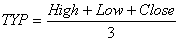Chart Pro online
User name (email):

 Recently used

 Forex

 Indices

# Typical Price (TYP)

Typical price (TYP) is technical analysis indicator which averages price for each period. Some technicians use it instead of closing price. Money flow index indicator uses typical price.

Typical price is calculated by formula:Typical Price (TYP)

Interpretation

Typical price is used by some technicians instead of closing price.

Example